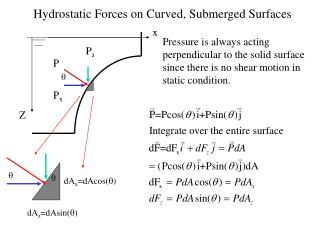DownloadDownload PresentationHydrostatic Forces on Curved, Submerged Surfaces

# Hydrostatic Forces on Curved, Submerged Surfaces

Télécharger la présentation## Hydrostatic Forces on Curved, Submerged Surfaces

- - - - - - - - - - - - - - - - - - - - - - - - - - - E N D - - - - - - - - - - - - - - - - - - - - - - - - - - -
##### Presentation Transcript

1. Hydrostatic Forces on Curved, Submerged Surfaces x Pressure is always acting perpendicular to the solid surface since there is no shear motion in static condition. Pz P q Px Z q q dAx=dAcos(q) dAz=dAsin(q)

2. Projected Forces x h dAz Z Integrated over all elements

3. Buoyancy Force acting down FD= rgV1 from Buoyancy = FU-FD =rg(V2-V1)=rgV V: volume occupied by the object Force acting up FU = rgV2 from

4. x Horizontal Forces h Projected area Ax dAz Finding Fx is to determine the force acting on a plane submerged surface oriented perpendicular to the surface. Ax is the projection of the curved surface on the yz plane. Similar conclusion can be made to the force in the y direction Fy. Z Integrated over all elements h dAx Equivalent system: A plane surface perpendicular to the free surface

5. Examples Determine the magnitude of the resultant force acting on the hemispherical surface. x 2 m R=0.5 m z equals minus

6. Line of Action Horizontal direction: line of action goes through z’ zC z’ Projection in x-direction Vertical direction: the line of action is 3R/8 away from the center of the hemisphere z The resultant moment of both forces with respect to the center of the hemisphere should be zero: Fx(2.03125-2)-Fz(0.1875) =15386(0.03125)-2564(0.1875)=0 location of the centroid for a hemisphere is 3R/8=0.1875(m) away from the equator plane